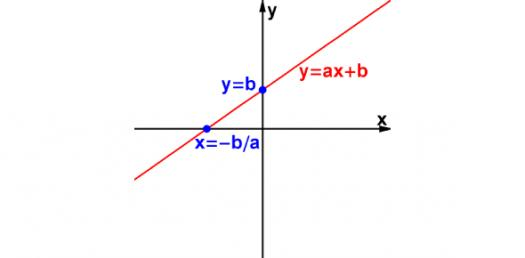# Math: Quiz On Linear Equation Word Problem!

10 Questions | Attempts: 466
ShareSettingsAre you any good with mathematical word problems? Give this quiz a try. A word problem in mathematics education is generally used to refer to any mathematical exercise in which an example consisting of words and numbers is given. The problem is given more in words, as opposed to mathematical notation. Taking this quiz will help you know about mathematical word problems.

• 1.
If Betsy has n quarters.  What expression could be used to find the total value of her coins in dollars?
• A.

25n

• B.

0.25 +n

• C.

25 + n

• D.

0.25n

• 2.
Jed reads p pages each day in a 200 page book.  What expression could be used to find the number days it would take to read the book?
• A.

200/p

• B.

200p

• C.

200 + p

• D.

200 - p

• 3.
Which verbal expression does NOT represent   9 - x
• A.

X less than nine

• B.

X decreased by nine

• C.

Subtract x from nine

• D.

The difference of nine and x

• 4.
Write "the product of 16 and n" as an algebraic expression.
• 5.
Which of the following verbal expressions does NOT translate into  n + 3 ?
• A.

• B.

A number plus 3

• C.

A number decreased by 3

• D.

3 more than a number

• 6.
Which of the following equations could be used to find the area of a rectangle with side lengths of 5cm and 7cm?
• A.

A = 5 + 5 + 7 + 7

• B.

A = 1/2 ( 5 )( 7 )

• C.

A = 5 + 7

• D.

A = (5)(7)

• 7.
Which phrase represents the expression 7x + 2 ?
• A.

Seven times two plus a number

• B.

Two times a number plus seven

• C.

Two less than a number multiplied multiplied by seven

• D.

Two plus a number multiplied by seven

• 8.
Write an algebraic expression for  " 18 less than a number x
• 9.
The state fair charges \$6 admission and \$2 for each ride.  Which expression could represent the total cost after admission is paid for one person and he/she rides "r" rides?
• A.

6 + 2r

• B.

2 + 6r

• C.

6 + 3r

• 10.
Which of the following equations represents the perimeter of a rectangle with sides L and W?
• A.

P = (L)(W)

• B.

P = L + W

• C.

P = 2L + 2W

• D.

P = (2L)(2W)

## Related TopicsBack to top
×

Wait!
Here's an interesting quiz for you.